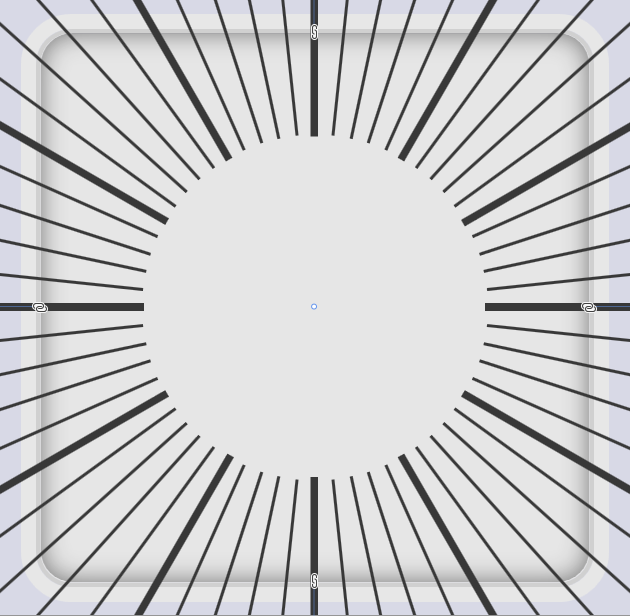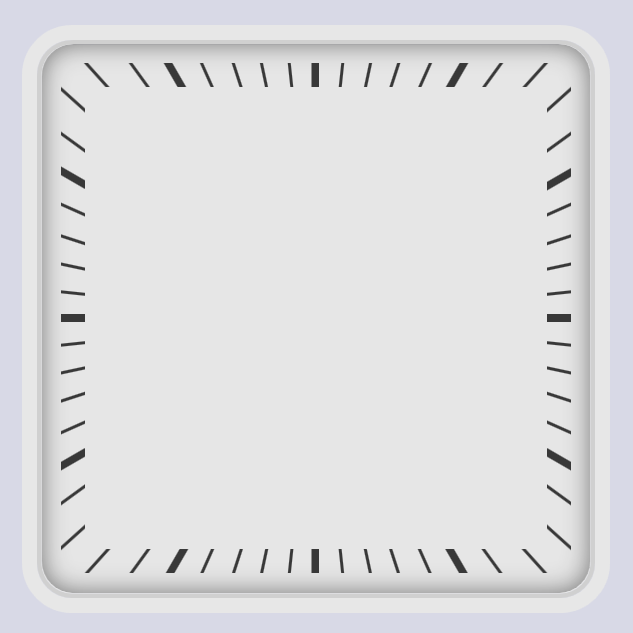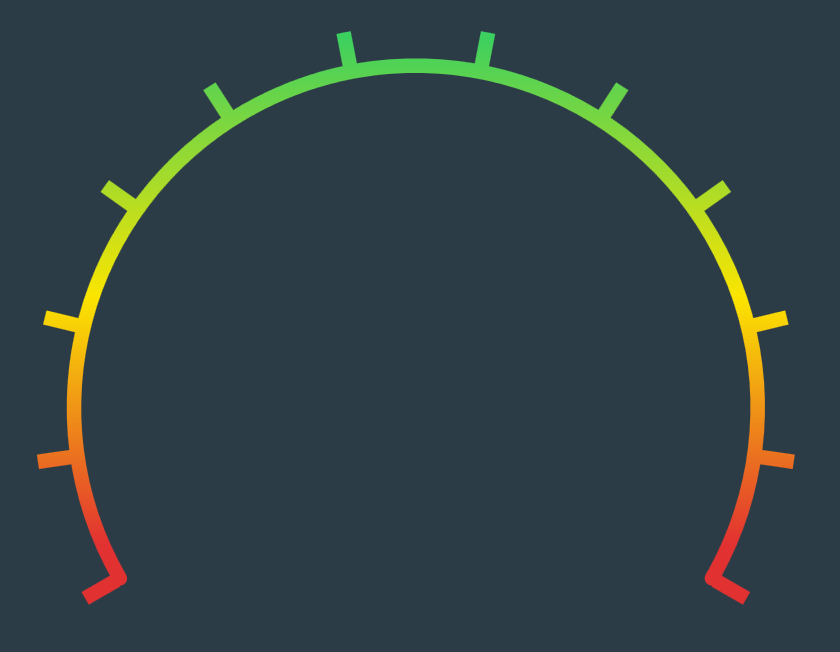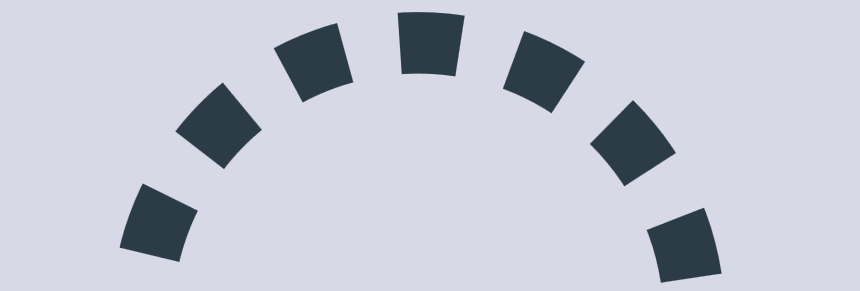# [WPF] 使用 HandyControl 的 CirclePanel 画出表盘刻度

## 1. 前言

double radius = BackEllipse.Width / 2;
double min = 0; double max = 100;
double step = 360.0 / (max - min);
for (int i = 0; i < max - min; i++)
{
Line lineScale = new Line
{
X1 = ((radius - 20) * Math.Cos(i * step * Math.PI / 180)) + radius,
Y1 = ((radius - 20) * Math.Sin(i * step * Math.PI / 180)) + radius,
X2 = (radius * Math.Cos(i * step * Math.PI / 180)) + radius,
Y2 = (radius * Math.Sin(i * step * Math.PI / 180)) + radius,
Stroke = Brushes.Red,
StrokeThickness = 2
};

}


## 2. 使用 CirclePanel 实现

<x:Array x:Key="AuthorList" Type="{x:Type sys:String}">
<sys:String>Mahesh Chand</sys:String>
<sys:String>Praveen Kumar</sys:String>
<sys:String>Raj Beniwal</sys:String>
<sys:String>Neel Beniwal</sys:String>
<sys:String>Sam Hobbs</sys:String>
</x:Array>


<ContentControl Content="111111111111">
<ContentControl.ContentTemplate>
<DataTemplate>
<ItemsControl ItemsSource="{Binding}">
<ItemsControl.ItemTemplate>
<DataTemplate>
<Rectangle Width="10"
Height="3"
Fill="#383838" />
</DataTemplate>
</ItemsControl.ItemTemplate>
</ItemsControl>
</DataTemplate>
</ContentControl.ContentTemplate>
</ContentControl>


<ItemsControl.ItemsPanel>
<ItemsPanelTemplate>
<hc:CirclePanel Diameter="310" />
</ItemsPanelTemplate>
</ItemsControl.ItemsPanel>## 3. 用 DataTrigger 实现不同的指针

<ContentControl Content="100001000010000100001000010000100001000010000100001000010000">


<DataTemplate>
<Rectangle x:Name="Tick"
Width="10"
Height="2"
Fill="#383838" />
<DataTemplate.Triggers>
<DataTrigger Binding="{Binding}" Value="1">
<Setter TargetName="Tick" Property="Height" Value="5" />
<Setter TargetName="Tick" Property="Width" Value="16" />
<Setter TargetName="Tick" Property="Margin" Value="0,0,3,0" />
</DataTrigger>
</DataTemplate.Triggers>
</DataTemplate>## 4. 用 OpacityMask 实现方形表盘<Border x:Name="Border"
Width="340"
Height="340"
BorderBrush="White"
BorderThickness="16" />
<ContentControl Style="{StaticResource ClockControl2}">
<ContentControl Margin="-100" Content="100001000010000100001000010000100001000010000100001000010000">
<VisualBrush Stretch="None" Visual="{Binding ElementName=Border}" />## 5. 用 ArcPanel 实现仪表盘

CirclePanel 虽然很好用，可惜的是不能实现弧形布局，于是我又另外找了 HeBianGu 的 ArcPanel 来实现仪表板，用它替换掉 CirclePanel 即可实现弧形布局的刻度线：

<ItemsControl.ItemsPanel>
<ItemsPanelTemplate>
<h:ArcPanel Width="200"
Height="200"
AngleToCenter="True"
EndAngle="-30"
StartAngle="210" />
</ItemsPanelTemplate>
</ItemsControl.ItemsPanel>
`## 5. 最后posted @ 2022-08-31 09:28  dino.c  阅读(2706)  评论(6编辑  收藏  举报